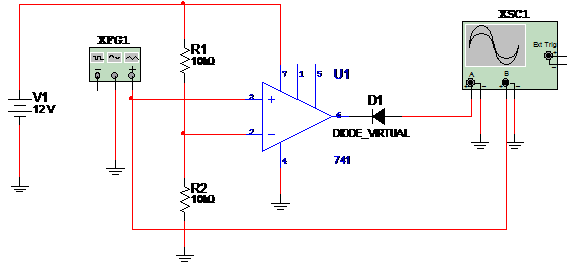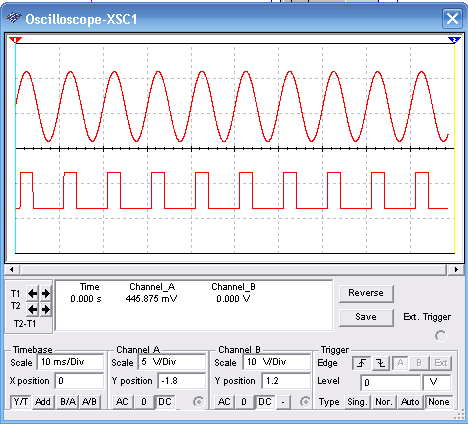### DatasheetsN° of component

## Comparator With Op. Amp. (MSB069E)

This simulation shows how to use an operational amplifier as a voltage comparator. The sample uses the 741 but it can be replaced with any other suitable op. amp. The power supply is simple (one voltage) and in the simulation a sinusoidal signal is applied to the input. The circuit for the simulation is shown in the figure 1.Figure 1 – The circuit

Trigger voltage is given by:

Vd = Vcc(R2/(R1+R2)) onde

Where:

Vd is the trigger voltage

Vcc is the supply voltage

R1 and R2 are the resistors;

Wave shapes in the circuit as displayed in the virtual oscilloscope of the Multisim are shown in figure 2.Figure 2 – Wave shapes in the circuit